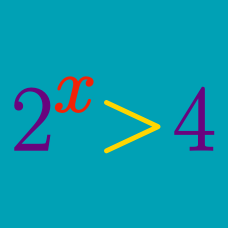Algebra

# Exponential Inequalities - Same Base

What is the range of $x$ that satisfies the inequality $2^{2x+4} < 2^{3x+8}?$

If $6^{a+b} < 6^{8}$ and $5^{b-a} = 5^{4},$ what is the range of $a?$

If $\frac{3^X}{3\cdot 3^Y} < 1,$ which of the following is true about $X$ and $Y?$

What is the range of $x$ that satisfies the inequality $3^{x+5} < 3^{7}?$

What is the range of $x$ that satisfies the inequality $2^x < 8?$

×